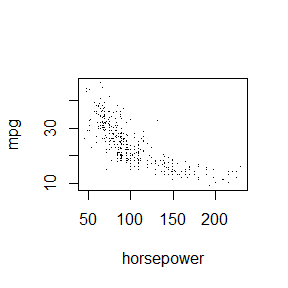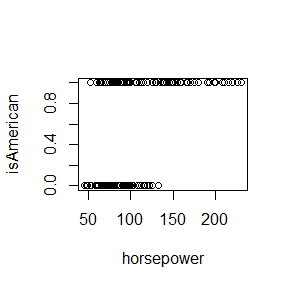# Informal tests of surveyCV using the Auto dataset

Informally test our various CV functions on the ISLR::Auto dataset using SRS-CV, clustered-CV, and stratified-CV.
(We are arbitrarily using year as either clusterID or stratumID.)

Just run the functions on a somewhat arbitrary dataset to ensure that the output format is what we’re looking for, and that the output is roughly consistent across the different functions that are doing the same thing.

• TODO: Add tests for a case with both clusters AND strata simultaneously.
• TODO: Add tests for cases with sampling weights and/or fpc’s.
• TODO: Add tests for transformed responses within formulae, eg “log(y) ~ …”
• TODO: Turn these into formal tests in the tests directory, not a vignette.
• TODO: Replace this vignette of informal tests with a practical demo vignette: how can you use surveyCV appropriately on a real complex-survey dataset?
library(surveyCV)
library(survey)
library(ISLR)
data(Auto)
with(Auto, plot(horsepower, mpg, pch = '.'))## cv.svy()

# Test the general cv.svy() function

# The first line's results are usually around:
# linear lm:      mean=24.3, se=1.45
cv.svy(Auto, c("mpg~poly(horsepower,1, raw = TRUE)",
"mpg~poly(horsepower,2, raw = TRUE)"),
nfolds = 10)
##            mean     SE
## .Model_1 24.071 1.8454
## .Model_2 19.173 1.7553
cv.svy(Auto, c("mpg~poly(horsepower,1,raw=TRUE)",
"mpg~poly(horsepower,2,raw=TRUE)"),
nfolds = 10, clusterID = "year")
##            mean     SE
## .Model_1 27.271 4.6104
## .Model_2 21.184 3.8695
cv.svy(Auto, c("mpg~poly(horsepower,1, raw = TRUE)",
"mpg~poly(horsepower,2, raw = TRUE)"),
nfolds = 10, strataID = "year")
##            mean     SE
## .Model_1 24.110 1.7364
## .Model_2 19.114 1.6673

## cv.svydesign()

# Test the cv.svydesign() function, which takes a svydesign object and formulas
auto.srs.svy <- svydesign(ids = ~0,
data = Auto)
## Warning in svydesign.default(ids = ~0, data = Auto): No weights or probabilities
## supplied, assuming equal probability
auto.clus.svy <- svydesign(ids = ~year,
data = Auto)
## Warning in svydesign.default(ids = ~year, data = Auto): No weights or
## probabilities supplied, assuming equal probability
auto.strat.svy <- svydesign(ids = ~0,
strata = ~year,
data = Auto)
## Warning in svydesign.default(ids = ~0, strata = ~year, data = Auto): No weights
## or probabilities supplied, assuming equal probability
cv.svydesign(formulae = c("mpg~poly(horsepower,1, raw = TRUE)",
"mpg~poly(horsepower,2, raw = TRUE)",
"mpg~poly(horsepower,3, raw = TRUE)"),
design_object = auto.srs.svy, nfolds = 10)
##            mean     SE
## .Model_1 24.272 1.8579
## .Model_2 19.276 1.7724
## .Model_3 19.378 1.8126
cv.svydesign(formulae = c("mpg~poly(horsepower,1, raw = TRUE)",
"mpg~poly(horsepower,2, raw = TRUE)",
"mpg~poly(horsepower,3, raw = TRUE)"),
design_object = auto.clus.svy, nfolds = 10)
##            mean     SE
## .Model_1 26.963 4.6224
## .Model_2 20.740 3.8867
## .Model_3 20.789 3.8934
cv.svydesign(formulae = c("mpg~poly(horsepower,1, raw = TRUE)",
"mpg~poly(horsepower,2, raw = TRUE)",
"mpg~poly(horsepower,3, raw = TRUE)"),
design_object = auto.strat.svy, nfolds = 10)
##            mean     SE
## .Model_1 24.041 1.7297
## .Model_2 19.108 1.6763
## .Model_3 19.130 1.7104

## cv.svyglm()

# Test the cv.svyglm() function, which takes one svyglm object
srs.model <- svyglm(mpg~horsepower+I(horsepower^2)+I(horsepower^3), design = auto.srs.svy)
clus.model <- svyglm(mpg~horsepower+I(horsepower^2)+I(horsepower^3), design = auto.clus.svy)
strat.model <- svyglm(mpg~horsepower+I(horsepower^2)+I(horsepower^3), design = auto.strat.svy)

cv.svyglm(glm = srs.model, nfolds = 10)
##           mean     SE
## .Model_1 19.43 1.8208
cv.svyglm(glm = clus.model, nfolds = 10)
##            mean     SE
## .Model_1 20.625 3.8178
cv.svyglm(glm = strat.model, nfolds = 10)
##            mean     SE
## .Model_1 19.279 1.7197

## Test for equivalence of the 3 functions at same random seed

seed = 20210708

set.seed(seed)
cv.svy(Auto, "mpg~horsepower+I(horsepower^2)+I(horsepower^3)",
nfolds = 10)
##            mean     SE
## .Model_1 19.466 1.8254
set.seed(seed)
cv.svydesign(formulae = "mpg~horsepower+I(horsepower^2)+I(horsepower^3)",
design_object = auto.srs.svy, nfolds = 10)
##            mean     SE
## .Model_1 19.466 1.8254
srs.model <- svyglm(mpg~horsepower+I(horsepower^2)+I(horsepower^3), design = auto.srs.svy)
set.seed(seed)
cv.svyglm(glm = srs.model, nfolds = 10)
##            mean     SE
## .Model_1 19.466 1.8254

## Test of logistic regression

Auto$isAmerican <- Auto$origin == 1
with(Auto, plot(horsepower, isAmerican))cv.svy(Auto, c("isAmerican~horsepower",
"isAmerican~horsepower+I(horsepower^2)",
"isAmerican~horsepower+I(horsepower^2)+I(horsepower^3)"),
nfolds = 10, method = "logistic")
##             mean     SE
## .Model_1 0.50118 0.0231
## .Model_2 0.50184 0.0233
## .Model_3 0.49620 0.0245

## Tests that we expect should fail

# Should stop early because method isn't linear or logistic
try(cv.svy(Auto, "mpg~horsepower+I(horsepower^2)+I(horsepower^3)",
nfolds = 10,
method = "abcde"))
## Error in match.arg(method) : 'arg' should be one of "linear", "logistic"
# Should try to run but crash because response variable isn't 0/1
try(cv.svy(Auto, "mpg~horsepower+I(horsepower^2)+I(horsepower^3)",
nfolds = 10,
method = "logistic"))
## Error in eval(family\$initialize) : y values must be 0 <= y <= 1